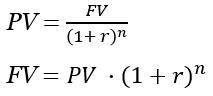Use this calculator to easily calculate the present value, future value, intereste rate or fixed payment. TVM calculator applicable to deposits, credits, investments, and others.

## How to use the TVM calculator

Our online calculator is a simple and easy to use tool to calculate various quantities related to the time value of money such as present value, future value, interest rate and repeating payment required to cover a loan or to increase a deposits value to a certain amount. After deciding what you want to compute for, provide the remaining values and press Calculate. Consult the question marks next to each fields label if you are uncertain on how to proceed.

As a general rule, enter values with a minus in front of them if they are cash outflows. For example, enter -100 if you are depositing or investing 100 USD. Enter just 100 if you are taking a loan worth \$100. Similarly, enter -20 if you are withdrawing money from an investment or deposit and enter 20 if you are depositing \$20 to cover the interest or the principal of a loan/credit.

Note that the input in the interest rate field in the calculator needs to be the effective interest rate based on the period for which you are performing the calculation. If the period is a year (e.g. youve entered 5 for the Number of periods field and this is a 5-year loan) then you should enter the effective annualized interest rate. If the period is a month, you should enter the effective monthly interest rate instead.

## Understanding the Time Value of Money

The powerful concept of time value of money reflects the simple fact that humans have a time preference: given identical gains, they would rather take them now rather than later. For example, if you can get \$10,000 now or in 5 years, youd choose to get them now, all other things being equal. This stems both from the ability to spend the money immediately (almost certain benefit) versus the uncertainty related to spending them in 5 years, eventually. Similarly, if you invest the money or put them in a bank deposit they can earn you interest during these 5 years: something you would not be able to do otherwise. Time-related opportunity costs are the reason the concept of time value of money is key in managing personal or business finances.

Time preference is the reason for interest rates to exist: they are in fact the price paid for using money in a given period of time. It compensates the depositor or lender for their opportunity cost. Consequently, interest rates are low when the perceived opportunity cost is low and high if they are high. Similarly, investors inform their decisions on whether to pass or get in a certain venture by calculating the expected return (increase in value of their capital) versus alternative investments.

## TVM Formula

The calculation of time value of money (TVM) depends on the following inputs: present value (PV), future value (FV), the value of the individual payments in each compounding period (A), the number of periods (n), the interest rate (r).

You can use the following two formulas to calculate present value and future value without periodical payments:If there are periodical payments they need to be adjusted similar to the present value / future value and added to the formulas above. Calculating the amount of the periodical payment required is a simple analytical transformation handled by the TVM solver automatically.

Calculating the number of periods or the interest rate however is not trivial as there is no analytical solution. It must therefore be done through successive approximation until a reasonably accurate value is pinpointed. Our time value of money calculator can easily do this for you.

## What can the TVM formula calculator solve for?

Making better wealth management decisions can become a bit easier with the help of our online TVM solver. Using the formulas above and their relevant transformations, the solver can calculate:

• present value
• future value
• number of periods
• required rate of interest
• the value of each payment in a compounding period

Additionally, more complex formulas can include a growing instead of fixed periodical payment (g), but this is not supported in the TVM calculator at the present.

## Financial caution

This is a simple online tool which is a good starting point in estimating different quantities related to an investment or credit, but is by no means the end of such a process. You should always consult a qualified professional when making important financial decisions and long-term agreements, such as long-term bank deposits. Use the information provided by the solver critically and at your own risk.

## Say hello

#### Find us at the office

Schwede- Busard street no. 40, 77937 Riyadh, Saudi Arabia

#### Give us a ring

Smith Waltimyer
+86 488 682 876
Mon - Fri, 10:00-17:00